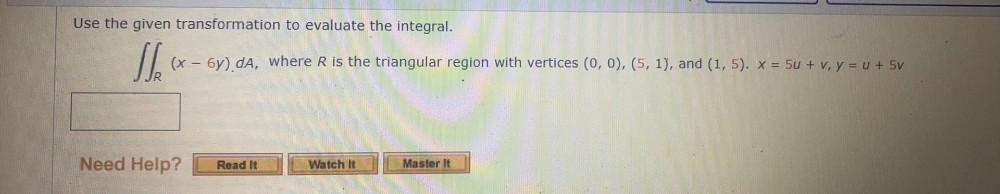Question:

# Use the given transformation to evaluate the integral. (x - 5y) da, where R is the triangular region with vertices (0,0), (5, 1)Use the given transformation to evaluate the integral. (x - 5y) da, where R is the triangular region with vertices (0,0), (5, 1), and (1,5). * = 5u + v, y = x + 5v Need Help? Read It Watch It Master it